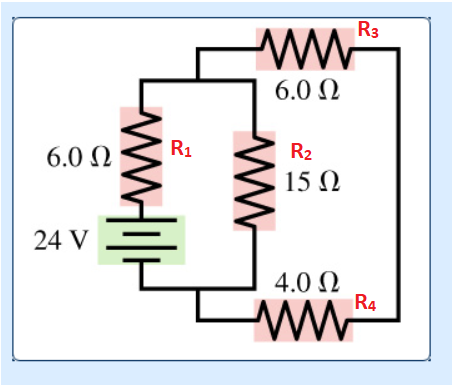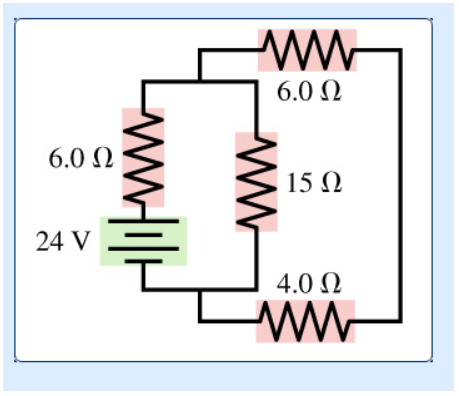# Problem: For the circuit shown in the figure, find the current through resistor R3 =6.0 Ω (the top one) and the potential difference across resistor R3=6.0 Ω

###### FREE Expert Solution

Equivalent resistance for resistors in parallel:

$\overline{)\frac{\mathbf{1}}{{\mathbf{R}}_{\mathbf{eq}}}{\mathbf{=}}\frac{\mathbf{1}}{{\mathbf{R}}_{\mathbf{1}}}{\mathbf{+}}\frac{\mathbf{1}}{{\mathbf{R}}_{\mathbf{2}}}{\mathbf{+}}{\mathbf{.}}{\mathbf{.}}{\mathbf{.}}{\mathbf{+}}\frac{\mathbf{1}}{{\mathbf{R}}_{\mathbf{n}}}}$

or for 2 resistors:

$\overline{){{\mathbf{R}}}_{{\mathbf{eq}}}{\mathbf{=}}\frac{{\mathbf{R}}_{\mathbf{1}}{\mathbf{R}}_{\mathbf{2}}}{{\mathbf{R}}_{\mathbf{1}}\mathbf{+}{\mathbf{R}}_{\mathbf{2}}}}$

Equivalent resistance for resistors in series:

$\overline{){{\mathbf{R}}}_{{\mathbf{eq}}}{\mathbf{=}}{{\mathbf{R}}}_{{\mathbf{1}}}{\mathbf{+}}{{\mathbf{R}}}_{{\mathbf{2}}}{\mathbf{+}}{\mathbf{.}}{\mathbf{.}}{\mathbf{.}}{\mathbf{+}}{{\mathbf{R}}}_{{\mathbf{n}}}}$

Ohm's law:

$\overline{){\mathbit{i}}{\mathbf{=}}\frac{\mathbf{V}}{\mathbf{R}}}$R3 and R4 are in series:

R34 = R3 + R4 = 6.0 + 4.0 = 10.0 Ω

R2 and R34 are in parallel:

R234 = (1/R2 + 1/R34)-1 = (1/15 + 1/10)-1 = 6.0 Ω

95% (81 ratings)###### Problem DetailsFor the circuit shown in the figure, find the current through resistor R3 =6.0 Ω (the top one) and the potential difference across resistor R3=6.0 Ω